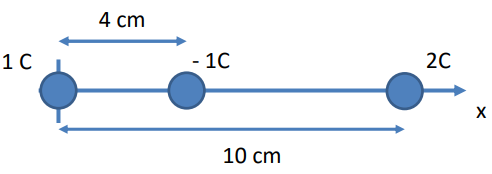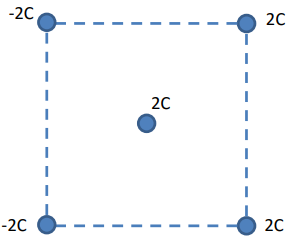Clutch Prep is now a part of Pearson
Ch 25: Electric Force & Field; Gauss' LawWorksheetSee all chapters

# Coulomb's Law (Electric Force)

See all sections
Sections
Electric Charge
Charging Objects
Charging By Induction
Conservation of Charge
Coulomb's Law (Electric Force)
Electric Field
Electric Fields in Capacitors
Electric Field Lines
Dipole Moment
Electric Fields in Conductors
Electric Flux
Gauss' Law

Concept #1: Coulomb's Law

Practice: If the force between two charges is F when the distance is d, what will the force between the two charges be if they were moved to a distance of 2d?

Example #1: Charges In A Line (Find Zero Force)

Practice: In which direction will the −1 C charge move? If it has a mass of 10 g, what will its initial acceleration be?Example #2: Charges In A Triangle (Rank Force Pairs)

Example #3: Charges in a Plane

Example #4: Exploiting Symmetry

Practice: What is the direction of the net force on the charge at the center of the square in the following figure?Example #5: Electroscope (Find Charge)